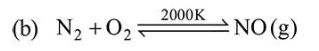# The correct statement with respect to dinitrogen is:

Question:

The correct statement with respect to dinitrogen is:

1. $\mathrm{N}_{2}$ is paramagnetic in nature.

2. it can combine with dioxygen at $25^{\circ} \mathrm{C}$.

3.  liquid dinitrogen is not used in cryosurgery.

4. it can be used as an inert diluent for reactive chemicals.

Correct Option: , 4

Solution:

(a) $\mathrm{N}_{2}$ is diamagnetic in nature.(c) Liquid $\mathrm{N}_{2}$ is used in cryosurgery.

(d) Because of its inertness, it is used where an inert atmosphere is required.